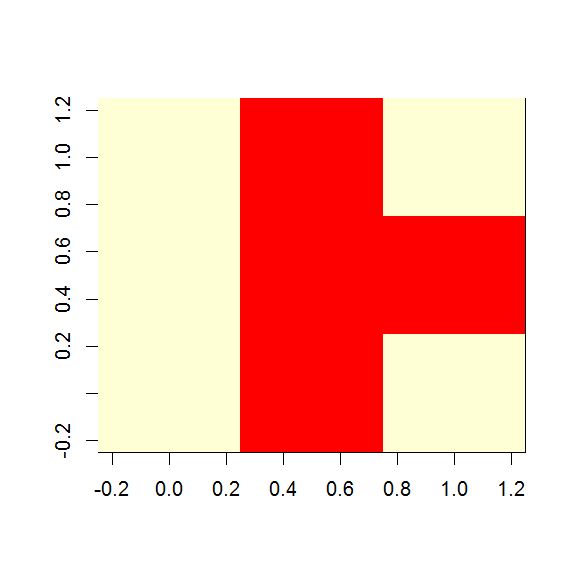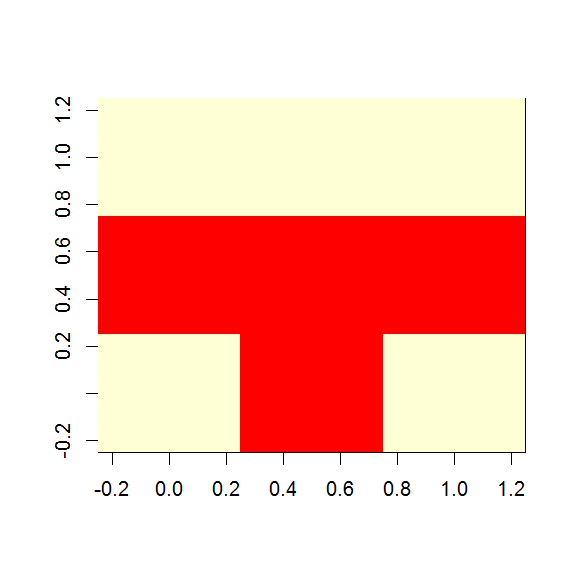Want to share your content on R-bloggers? click here if you have a blog, or here if you don't.

You have a matrix in R, and you want to visualise it – say, for example, with each cell coloured according to the value in the cell.  Not a heatmap, per se, as that requires clustering; just a simple, visual image.

Well, the answer is image() – however, there is the slightly bizarre coding choice buried in the help:

Notice that `image` interprets the `z` matrix as a table of `f(x[i], y[j])` values, so that the x axis corresponds to row number and the y axis to column number, with column 1 at the bottom, i.e. a 90 degree counter-clockwise rotation of the conventional printed layout of a matrix.

Let’s look at that:

```
mat <- matrix(c(1,1,1,0,0,0,1,0,1), nrow=3, byrow=TRUE)
mat
[,1] [,2] [,3]
[1,]    1    1    1
[2,]    0    0    0
[3,]    1    0    1

image(mat)
```

This produces:We can clearly see the 1,0,1 row is now the right-hand column i.e. the matrix has in fact been rotated 90 degrees anti-clockwise.

To counteract this we need to rotate the input matrix clockwise before passing to image:

```rotate <- function(x) t(apply(x, 2, rev))

image(rotate(mat))
```

This now produces what we might expect:Easy